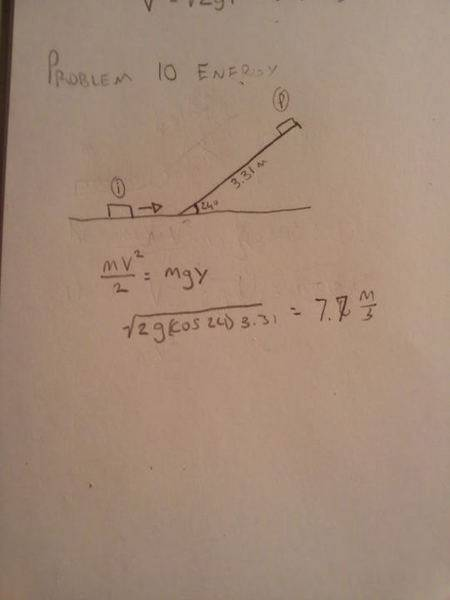# Velocity for a puck up a ramp

• talaroue

## Homework Statement

What minimum speed does a 136.4 g puck need to make it to the top of a 3.31-m-long, 24° frictionless ramp?

K=mV^2/2 U=mgY

## The Attempt at a Solutionthe 'y' in your equation is the vertical distance, not horizontal. So the cos24 should be sin24

wrong its cos.

cos angle = b/c

f * s + potential energy = mv^2 / 2

edit: mistake if you want height then its sin.

if the equation is mgh = mv^2/2 then you can cancel out the mass.

gh = v^2/2

9.82 * 1.35m = v^2 / 2

26.5 =v^2

v = 5.15m/s

but it think this is totally wrong.

You have the angle, hypotenuse and want to know the opposite side. Therefore you need to use the sine.

ahhhhh. Thanks guys i see where i went wrong you guys are awesome!